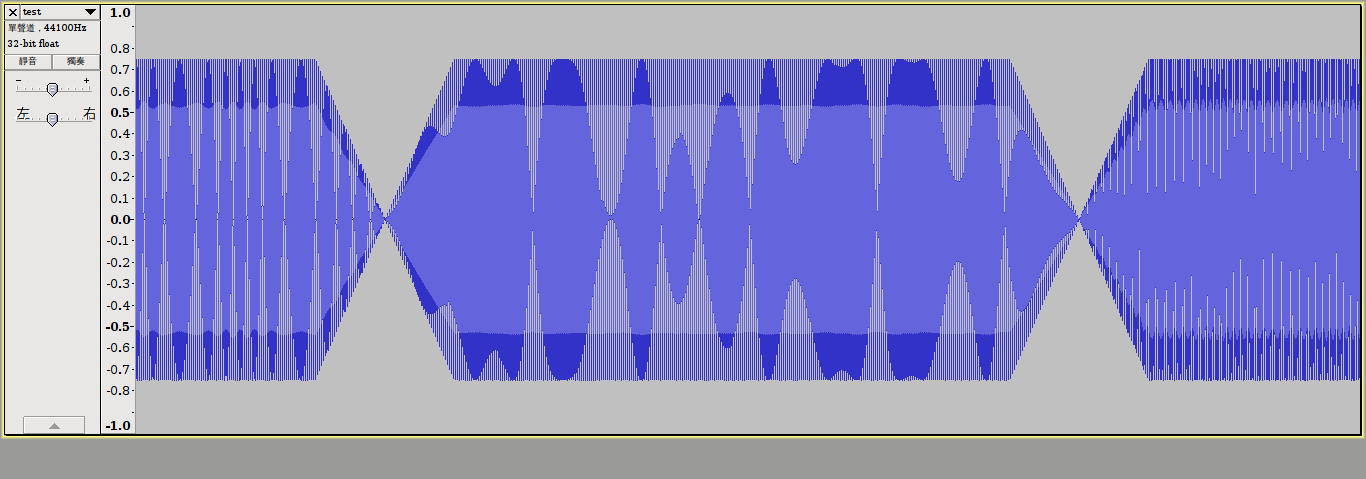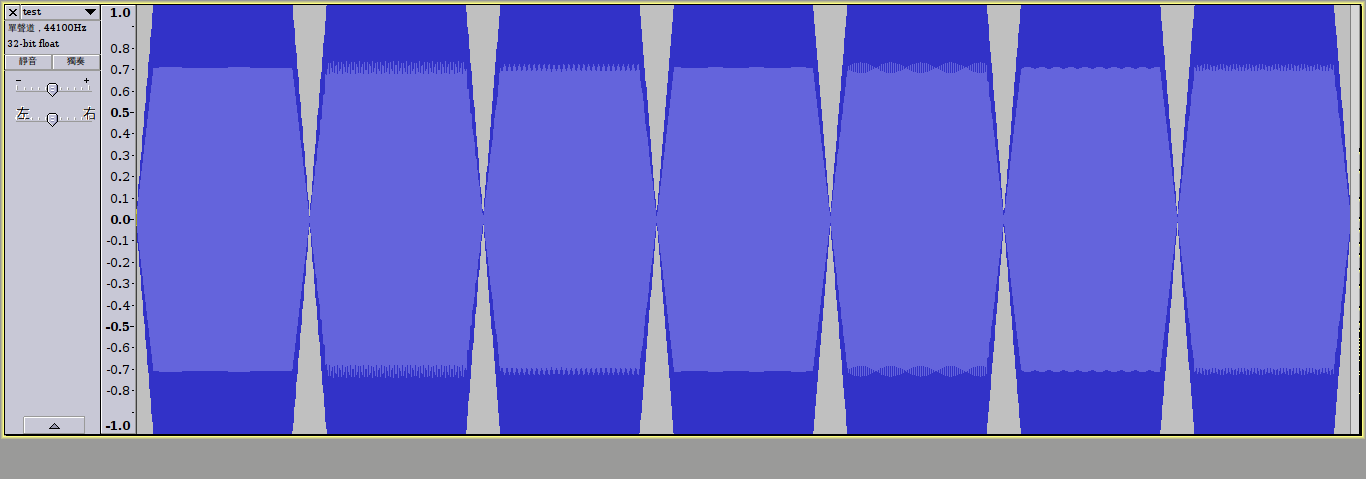Csound程式：

```		instr 1
;origin
;p4=pitch
;p3=duration
iamp = 100
ifreq = cpspch(p4)           ;convert pitch to frequent

av linen iamp, 0.1, p3, 0.1  ;ring the amplitude
a1 oscil av, ifreq, 1        ;oscillate f1
out a1                       ;output
endin```

### 顫音(Tremolo)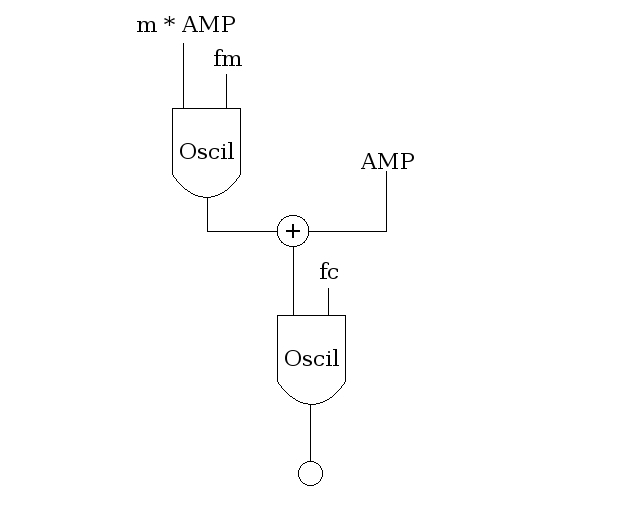```		instr 2
;tremolo
;p4=pitch
;p3=duration
iamp = 75
im = 0.25
ifm = 8
ifc = cpspch(p4)

aenv    linen  iamp, 0.1, p3, 0.1
amod    oscili  aenv*im, ifm, 2
asig    oscili  aenv+amod, ifc, 1
out     asig
endin```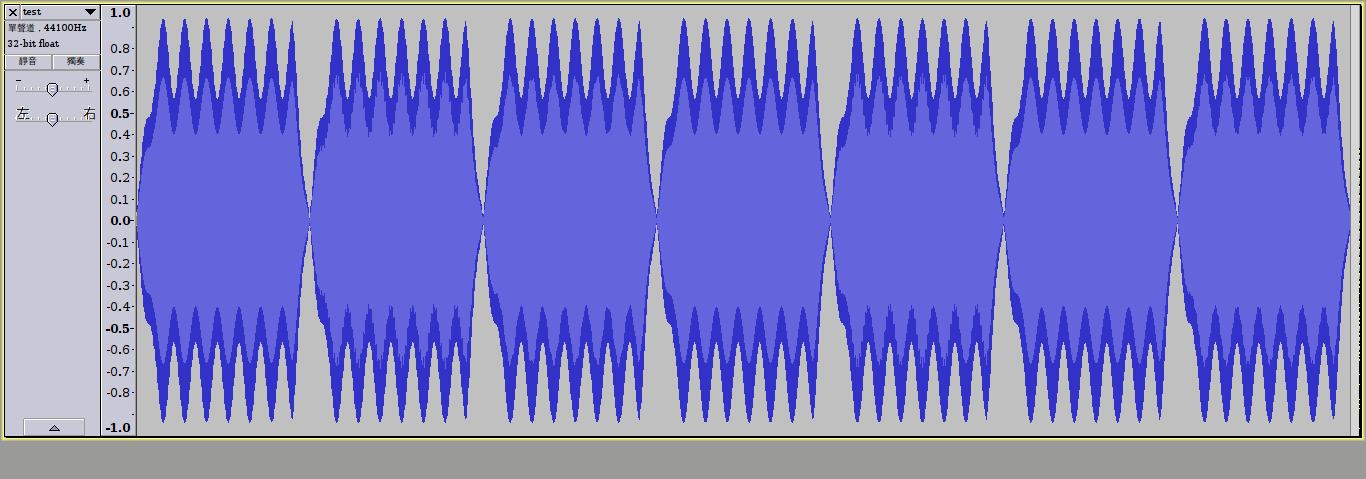### 抖音(Vibrato)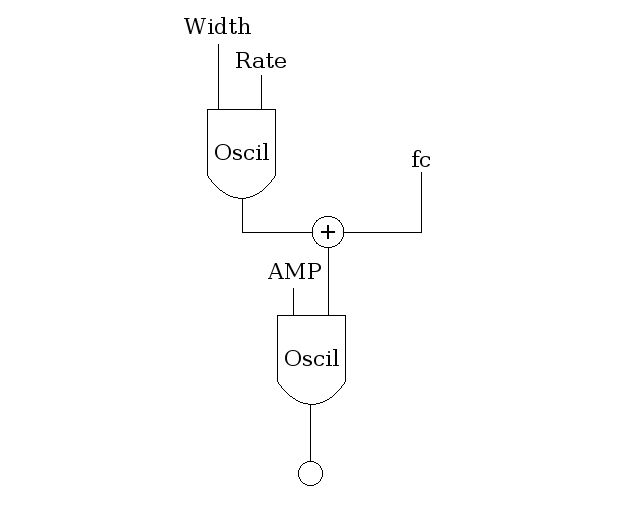```		instr 3
;vibrato
;p4=pitch
;p3=duration
iamp = 75
irate = 6
iwidth = 0.1
ifc = cpspch(p4)

aenv    linen  iamp, 0.1, p3, 0.1
amod    oscili  aenv*iwidth, irate, 2
asig    oscili  aenv, ifc+amod, 1
out     asig
endin```